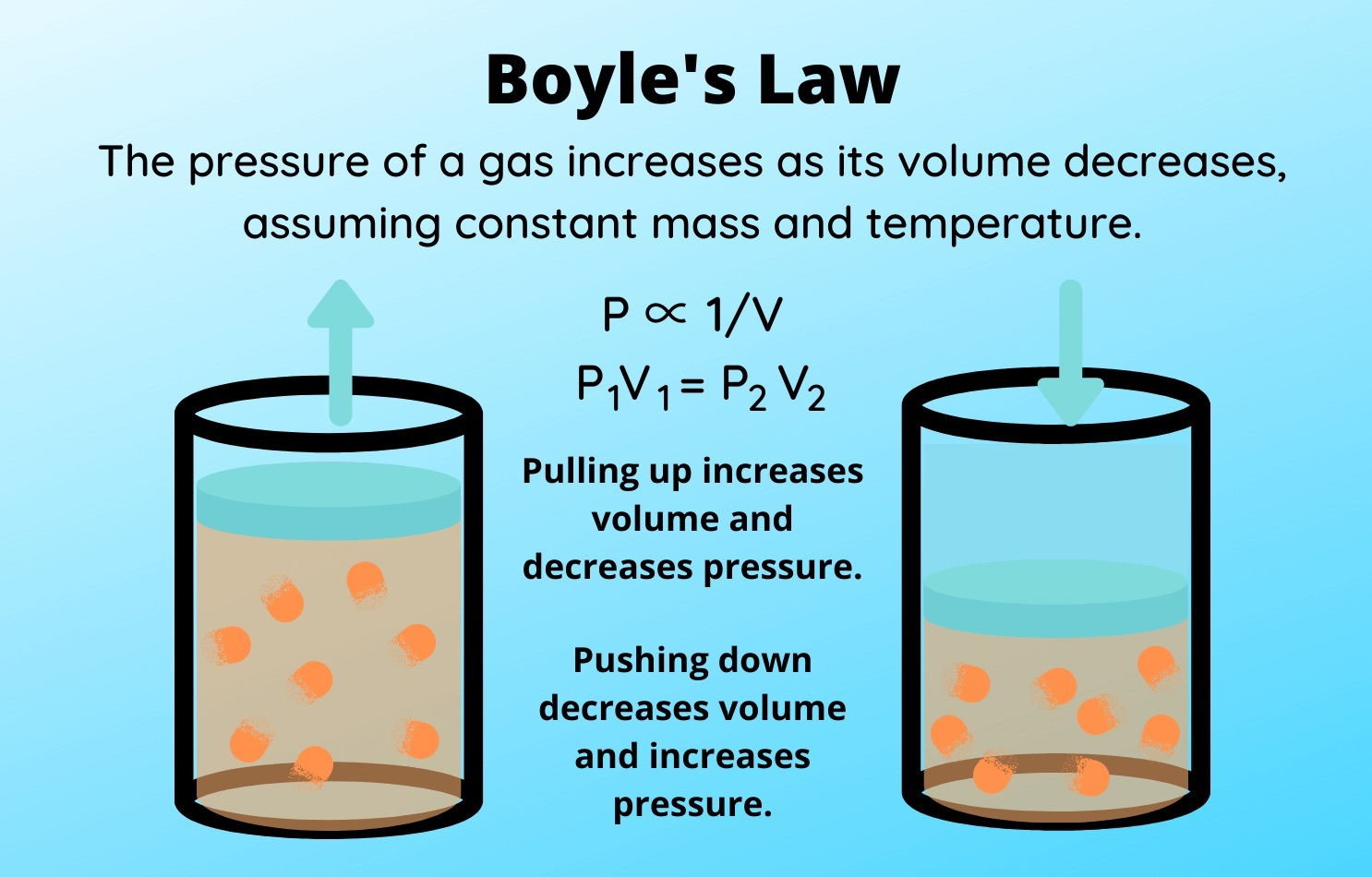## Latest Facts

### 14 Extraordinary Facts About Celandine PoppyMariejeanne Muncy

Published: 08 Sep 2023Source: Sciencenotes.org

Boyle’s Law is a fundamental principle in the field of chemistry that describes the relationship between the pressure and volume of a gas. Named after the Irish physicist Robert Boyle, this law has played a significant role in our understanding of the behavior of gases and has wide-ranging applications in various scientific and industrial fields.

In this article, we will explore 11 fascinating facts about Boyle’s Law, shedding light on its historical significance, underlying principles, and practical applications. From its early formulation to its use in modern gas laws, Boyle’s Law continues to captivate scientists and students alike with its profound insights into the behavior of gases. So, let’s delve into the intriguing world of Boyle’s Law and uncover some intriguing facts that will surely enhance your understanding of this essential concept in the realm of chemistry.

## Boyle’s Law is named after physicist Robert Boyle.

Rober Boyle, an Irish-born scientist, is credited with discovering the fundamental relationship between the pressure and volume of a gas.

## Boyle’s Law states that the pressure of a gas is inversely proportional to its volume when temperature is held constant.

This means that if you decrease the volume of a gas, its pressure will increase, and vice versa, as long as the temperature remains constant.

## Boyle’s Law is expressed by the equation P1V1 = P2V2.

This equation demonstrates the relationship between the initial pressure (P1) and volume (V1) of a gas, and the final pressure (P2) and volume (V2) after changes are made.

## Boyle’s Law can be applied to various real-life scenarios.

For example, when you inflate a balloon by blowing air into it, you are decreasing the volume of the air inside the balloon, which increases its pressure and causes it to expand.

## Boyle’s Law is a fundamental principle in the field of gas laws.

It serves as the basis for understanding the behavior of gases in various scientific and industrial applications.

## Boyle’s Law can be graphically represented by a pressure-volume curve.

When you plot the pressure and volume values of a gas on a graph, the relationship described by Boyle’s Law appears as a curved line.

## The temperature of a gas does not affect Boyle’s Law.

As long as the temperature remains constant, Boyle’s Law holds true for any gas.

## Boyle’s Law is a key concept in scuba diving.

Scuba divers must understand Boyle’s Law to prevent decompression sickness, also known as the bends, which can occur when ascending too quickly due to changes in pressure.

## Boyle’s Law can be used to calculate the volume of a gas at different pressures.

By rearranging the equation, you can solve for volume given the initial and final pressures of a gas.

## Boyle’s Law is part of the ideal gas law.

The ideal gas law combines Boyle’s Law with two other gas laws—Charles’ Law and Avogadro’s Law—and relates the pressure, volume, temperature, and number of moles of a gas.

## Boyle’s Law provides a foundation for understanding the behavior of gases in weather systems.

It helps meteorologists analyze how changes in pressure affect atmospheric conditions such as air mass movements and the formation of weather patterns.

## Conclusion

Boyle’s Law is an essential concept in the field of chemistry, explaining the relationship between pressure and volume of a gas at constant temperature. Understanding this law is crucial for various applications, from scuba diving to industrial processes.

With its simple yet powerful equation, Boyle’s Law has provided numerous insights into the behavior of gases and has practical implications in everyday life. By studying this fundamental concept, scientists and engineers are able to design and optimize processes to maximize efficiency and safety.

From its initial formulation by Robert Boyle in the 17th century to its continued relevance in modern science, Boyle’s Law has continued to captivate researchers and enthusiasts alike. Its principles have expanded our understanding of gas behavior and paved the way for advancements in various scientific and technological fields.

As we continue to explore the intricacies of Boyle’s Law and other gas laws, we uncover the fascinating world of chemistry and gain a deeper appreciation for the interplay between laws, principles, and the physical world around us.

## FAQs

Q: What is Boyle’s Law?

A: Boyle’s Law states that at a constant temperature, the volume of a given amount of gas is inversely proportional to the pressure applied to it.

Q: Who discovered Boyle’s Law?

A: Boyle’s Law was formulated by the Irish scientist Robert Boyle in the 17th century.

Q: How is Boyle’s Law relevant in everyday life?

A: Boyle’s Law is applicable to various situations, such as using scuba diving equipment, inflating balloons, and even breathing. Understanding this law helps us comprehend the behavior of gases in these scenarios.

Q: Are there any limitations to Boyle’s Law?

A: Boyle’s Law assumes that the temperature remains constant, and it applies primarily to ideal gases. Real gases may exhibit deviations from the ideal behavior under certain conditions.

Q: What is the mathematical equation for Boyle’s Law?

A: The mathematical equation for Boyle’s Law is expressed as PV = constant, where P represents the pressure, V denotes the volume, and the product of pressure and volume remains constant at a given temperature.